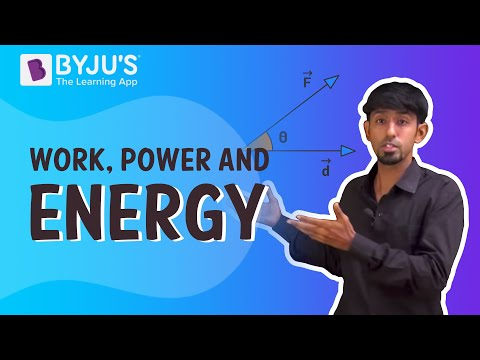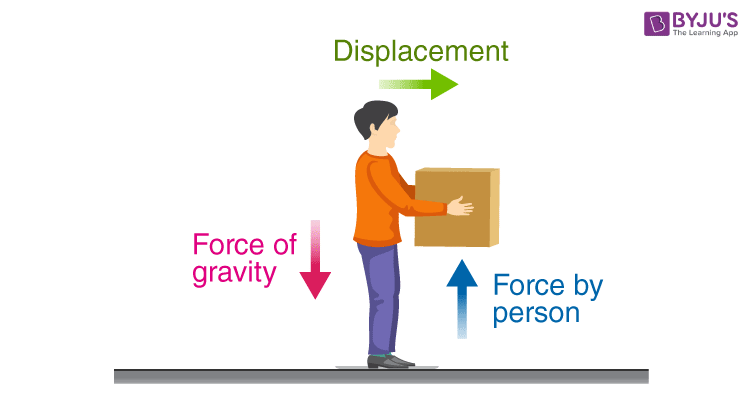# Introduction to Work DoneWhen we push a block with some force ‘F’ the body moves with some acceleration or in other words its speed increases or decreases depending on the direction of the force. As the speed increases or decreases, the kinetic energy of the system changes. We know energy can neither be created nor be destroyed so the energy must be getting transformed into some other form. In this case that is termed as work done. The energy decreases when negative energy is done and increases when positive work is done. Now we will see how to calculate work done. We already stated that for the above example:

Work done by force (F) = change in kinetic energy

$$\begin{array}{l}W = \frac{1}{2mv^2}~-~ \frac{1}{2mu^2}\end{array}$$
$$\begin{array}{l}W = \frac{1}{2m}~ (v^2 ~–~ u^2)\end{array}$$

Using,

$$\begin{array}{l}v^2~ –~ u^2 = 2as\end{array}$$
$$\begin{array}{l}W = \frac{1}{2}~ m~ (2as)\end{array}$$
$$\begin{array}{l}W = (ma).s\end{array}$$

From Newton’s second law,

$$\begin{array}{l}F = ma\end{array}$$
$$\begin{array}{l}W = F.s\end{array}$$

Where,

$$\begin{array}{l}F = \,\, force \,\, applied\end{array}$$
$$\begin{array}{l}s = displacement \,\, in \,\, the \,\, direction \,\, of \,\, force\end{array}$$

So can there be a situation when force is acting on a body and it is being displaced, but still the work done is zero? Let’s consider the example of a coolie carrying luggage. The force acting on the luggage is a force of gravity, which is vertical and displacement is horizontal. So the displacement in the direction of the force is zero, hence work done by gravity as well as the person is zero.Similarly, what is the energy when we are pushing a wall? It is very shocking that even though you feel fatigue but work done by you on the wall is zero. The displacement of the wall is zero. So according to the formula,

$$\begin{array}{l}W = F.s\end{array}$$
$$\begin{array}{l}W = F.0\end{array}$$
$$\begin{array}{l}W = 0\end{array}$$

## The below video helps to revise the concepts in the chapter Work and Energy Class 9So now that we know about work we will how it is related to power and much more. Just get in touch with BYJU’S – The learning app and fall in love with learning.

Test Your Knowledge On Work Done!